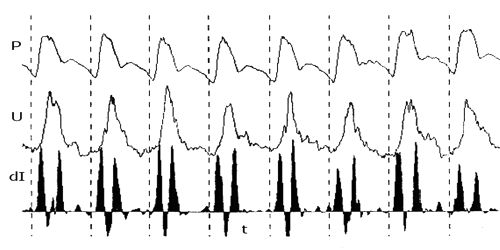Physics

# Intensity of WaveThe intensity of wave: The amount of energy transferred per second through unit area normal to the wave is called intensity of the wave. Wave intensity is the average power that travels through a given area as the wave travels through space. The intensity of sound waves is measured using the decibel scale. It is also called energy current or energy flux of the medium. It is denoted by I.

Intensity of wave, I = [energy density x velocity of the wave].Mathematically, it can be shown that,

I = 2ρπ2a2n2v … … … (1)

Here, ρ = density of the medium

n = frequency of the wave,

a = amplitude of the wave,

v = velocity of the wave.

From the above equation, it is found that,

I ∞ a2

= Ka2, here K is a constant.

i.e., Intensity is proportional to the square of amplitude.

In S.I. system, the unit of I is Jm-2s-1 or Wm-2.

So, Intensity of waves is defined as the power delivered per unit area. The unit of Intensity will be W.m-2. The wave energy comes from the simple harmonic motion of its particles. The total energy will equal the maximum kinetic energy.